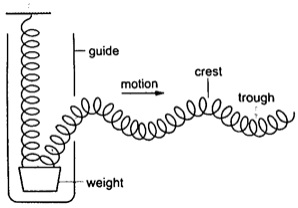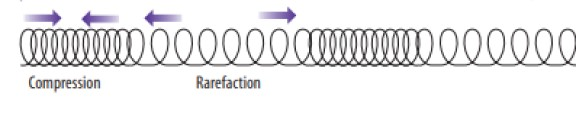# Waves I

### Guest Account

Attempt Form Two Physics Questions
Guest Account

# Waves I

- A wave is simply a disturbance that moves through a medium. Other waves do not require a medium to travel i.e. they can travel in a vacuum, are known as electromagnetic waves e.g. radio, X-rays, gamma rays UV rays etc.
- Other waves require a material medium to be transferred and are called mechanical waves i.e. water, sound waves etc.

### Transverse and longitudinal pulses and waves

#### 1. Transverse waves

– They consist of a crest and a trough.
- In this case the displacement of the medium caused by these pulses are perpendicular to the direction in which the wave (disturbance) travels.
- A pulse is a single non-repeated disturbance.
- If the pulses are repeated periodically (regularly) they produce a series of waves called periodic transverse wave train.
- They can be produced as shown below.#### 2. Longitudinal waves

- These are waves whereby the particles of the medium vibrate parallel to the direction of movement of the disturbance.
- When several turns of a spring are pulled together (compression) and then released they tend to spread out to their original position.
- When pulled apart (rarefaction) they also turn to their original position.
- In this case the displacement of the spring is parallel to the motion of the wave and this is known as longitudinal.### Characteristics of waves

1. All waves have speed which depends on the nature of disturbance.
2. All waves have wavelength (distance between two successive points in a wave). Represented by the symbol λ and is measured in metres.
3. All waves have frequency - ' f ' which is the number of waves passing a point in one second. It is measured in cycles per second or hertz (Hz). The period of a wave is the time required for a complete wave to pass a given point.
Therefore T = 1 / f or f = 1 / T (period is measured in seconds).
The speed 'v' is given as: v = λ / T, since f = 1 / T then v = (1 / T) x λ = f λ or v = f λ. This is the wave equation.
4. All waves have amplitude which is the maximum displacement of the particles of the medium as the wave passes.
Example 1
1. A rope is displaced at a frequency of 3 Hz. If the distance between two successive crests of the wave train is 0.8 m, calculate the speed of the waves along the rope.
Solution
v = f λ = 3 x 0.8 = 2.4 m
Hz = 2.4 m/s.

Example 2
2. The figure below illustrates part of the displacement-time graph of a wave travelling across water at a particular place with a velocity of 2 ms-1 Calculate the wave's:
a) Amplitude
b) Frequency (f)
c) Wavelength (λ)Solution
a) From the graph, maximum displacement
(a) = 0.4 cm.
b) From the graph, period T = time for one cycle = 0.20 seconds.
So f = 1 / T = 1 / 0.20 = 5 Hz.
c) Velocity = f λ hence λ = 2 / 5 = 0.4 m.